# SSAT Middle Level Math : Sets

## Example Questions

1 2 4 Next →

### Example Question #31 : How To Find The Missing Part Of A List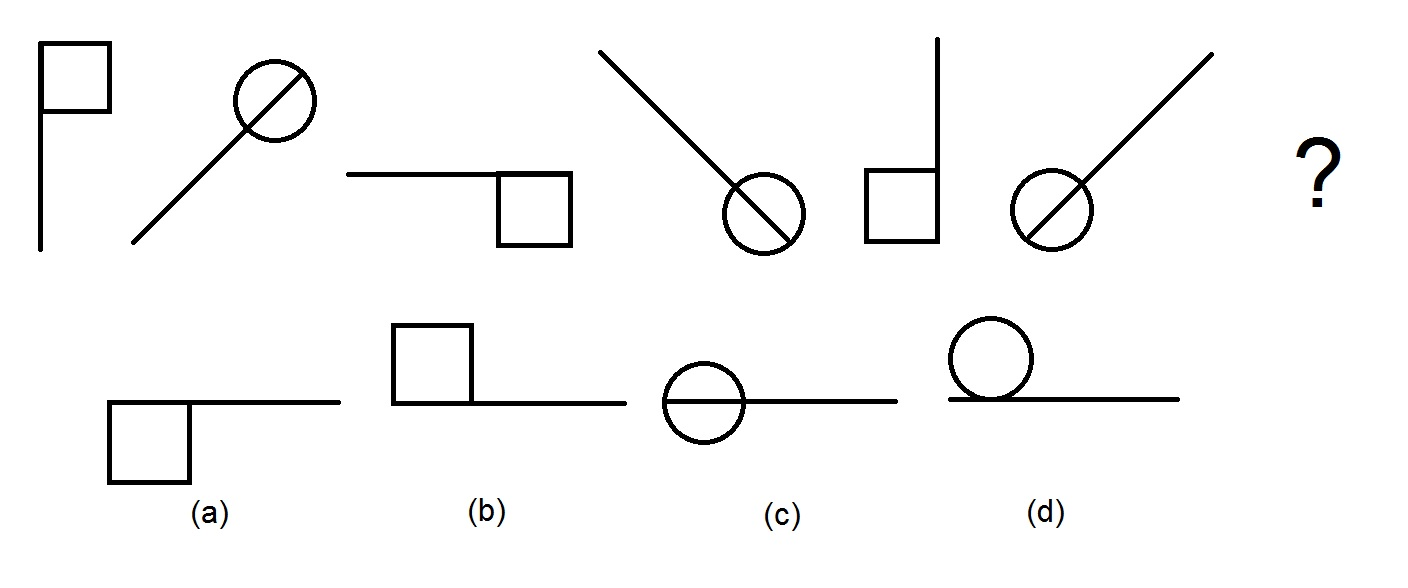Refer to the above diagram. The top row gives a sequence of figures. Which figure on the bottom row comes next?

Figure (d)

Figure (b)

Figure (a)

Figure (c)

Figure (b)

Explanation:

The figures alternate between lines with squares and lines with circles, so the next figure must be a line with a square. This eliminates Figures (c) and (d) and leaves Figures (a) and (b).

If we only look at every other figure - the ones with the squares - we note that each figure is the previous one rotated one quarter turn clockwise. The next figure in the sequence is therefore the figure resulting from a one-quarter turn of the fifth figure - this is Figure (b).

### Example Question #191 : Data Analysis And Probability

What number replaces the square in the sequence below?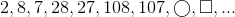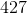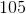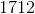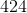Explanation:

The way to get from one entry of the sequence to the next alternates between multiplying by four and subtracting one, as follows: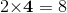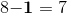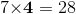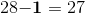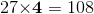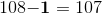Continuing the pattern: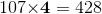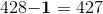, the correct choice.

### Example Question #33 : Sets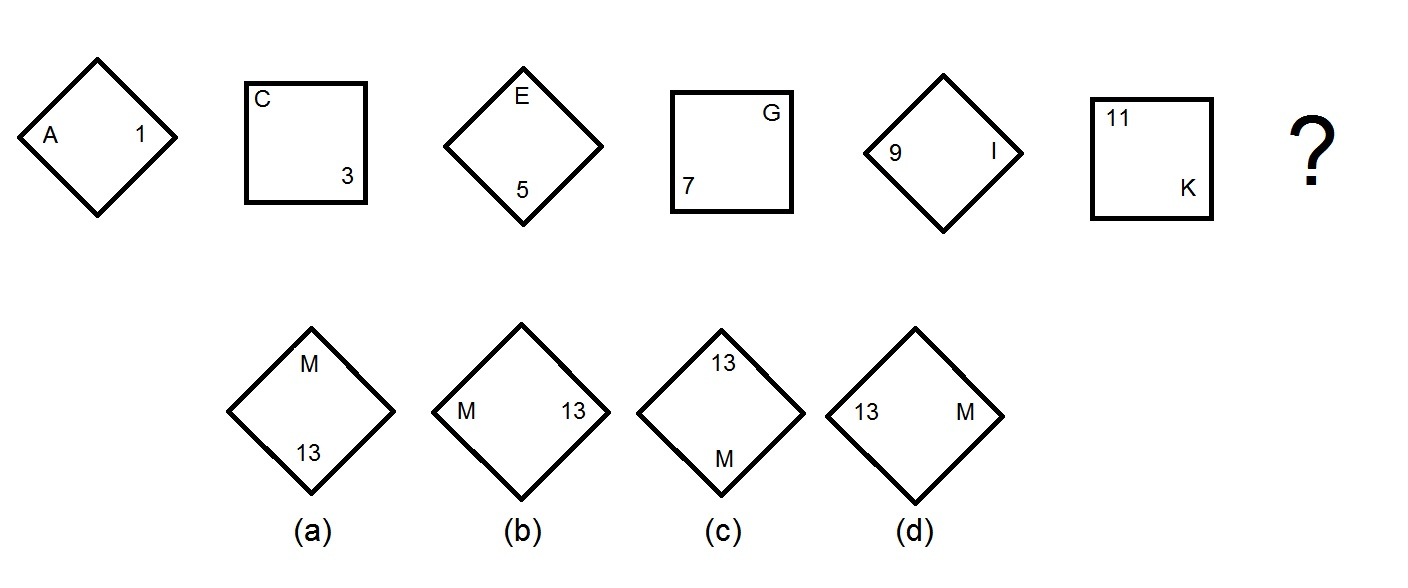On the top row in the above diagram is a sequence of figures. Which of the figures in the bottom row is the next one in the sequence?

Figure (b)

Figure (d)

Figure (c)

Figure (a)

Figure (c)

Explanation:

Each figure is obtained by rotating the previous figure one-eighth of a turn clockwise, with the position of the letter and the number also so changed. Also, the number increases by two, and the letter, which is always in the opposite corner, is the letter corresponding to the number (A = 1, C = 3, etc...).

The next number in the pattern is 13; the thirteenth letter in the alphabet is "M"; and, to continue the pattern of rotating one-eighth of a turn clockwise each time, the "M" must be at the very bottom, with the "13" being at the top. This means that Figure 3 is the next figure in the sequence.

1 2 4 Next →

### All SSAT Middle Level Math Resources